# Solution assignment 10 Linear functions and graphs

### Assignment 10

The linegoes through the points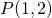and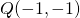. For which value of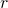is the line: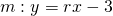parallel to?

### Solution

Parallel lines have equal slopes. The slope ofis: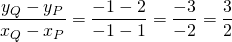The equation ofis: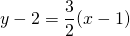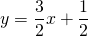The lineis parallel to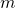if: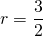and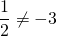Both conditions are satisfied and thus the lineis parallel toand does not coincide with it.